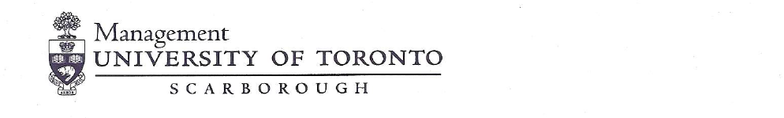# MGEB12H3 Lecture Notes - Lecture 1: Variance, Standard Deviation, Statistical Hypothesis Testing

49 views7 pages
School
UTSC
Department
Economics for Management Studies
Course
MGEB12H3
ProfessorMGEB12: Quantitative Methods in Economics-II
Problem Set-4
Chapter 11: 2, 10, 14, 18
Supplemental
1. In a hypothesis test for population variance, the hypotheses are:
100:
100:
2
1
2
H
Ho
If the sample size is 15 and the test is at 10% significance level, the rejection area will be:
A. 064.21,790.7 22
B. 685.23,571.6 22
C. 307.22,547.8 22
D. 996.24,261.7 22
E. 173.20,671.9 22
2. An assembly line produces widgets with a mean weight of 10 and a standard deviation of 0.200. A new
process supposedly will produce widgets with the same mean and a smaller standard deviation. A sample of 20
widgets produced by the new method has a sample standard deviation of 0.126. At a significance level of 10%,
can we conclude that:
A. The variance has not declined because the observed variance is less than 0.057 (The critical value.)
B. The variance has declined because the observed variance is less than 0.0245 (The critical value.)
C. The variance has not declined because the observed variance is less than 0.0245 (The critical value.)
D. The variance has declined because the observed variance is less than 0.066 (The critical value.)
E. None of the above
3. We are given the following information about two samples:
Sample A Sample B
S2 16.75 5
n 11 9
We want to test the hypothesis that population A has a larger variance than population B. The p-value is
approximately?
Unlock document

This preview shows pages 1-2 of the document.
Unlock all 7 pages and 3 million more documents.

2
A. 0.10
B. 0.05
C. 0.025
D. 0.01
E. None of the above
4. We are interested in determining whether or not the variances of the sales at two small grocery stores are
equal. A sample of 16 days of sales at each store indicated the following.
Store A Store B
nA = 16 nB = 15
SA = \$130 SB = \$100
Are the variances of the populations (from which these samples came) equal?
A. p-value < 0.05; reject H0
B. p-value > 0.10; do not reject H0
C. 0.05 < p-value < 0.10; do not reject H0
D. 0.025 < p-value < 0.05; reject H0
E. p-value > 0.10; do not reject H0 p-value < 0.01; reject H0
5. In a hypothesis test for the population variance, the hypotheses are 175: 2
0
H vs. 175: 2
1
H. If the
sample size is 25 and the test is being carried out at the 5% level of significance, the rejection region will be:
A. 2
< 15.6587 or 2
> 33.1963
B. 2
<12.4011 or 2
>39.3641
C. 2
< 16.4734 or 2
>34.3816
D. 2
< 13.1197 or 2
<37.6525
E. 2
< 11.2312 or 2
<39.7231
6. As its degrees of freedom increase, the chi-squared distribution approaches the shape of the:
A. Student t - distribution
B. Poisson distribution
C. Binomial Distribution
D. Normal distribution
E. F- Distribution
7. Last year, the standard deviation of the ages of the students at UTSC was 1.8 years. Recently, a sample of 61
students had a standard deviation of 2.1 years. We are interested in testing to see if there has been a significant
change in the standard deviation of the ages of the students at UTSC. The p-value for this test is
A. 0.05
B. between 0.05 and .10
C. between .05 and .01
D. 1.96
E. None of the above
8. We are interested in determining whether or not the variances of the sales at two music stores (A and B) are
equal. A sample of 26 days of sales at store A has a sample standard deviation of 30 while a sample of 16 days
of sales from store B has a sample standard deviation of 21. The p-value for this test is:
Unlock document

This preview shows pages 1-2 of the document.
Unlock all 7 pages and 3 million more documents.